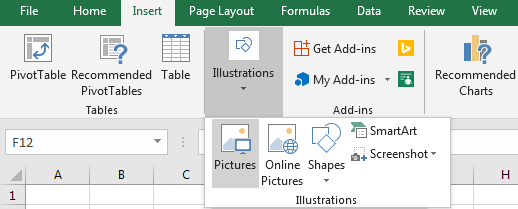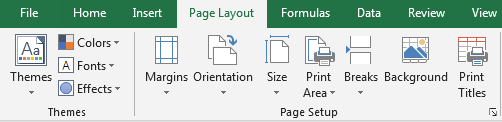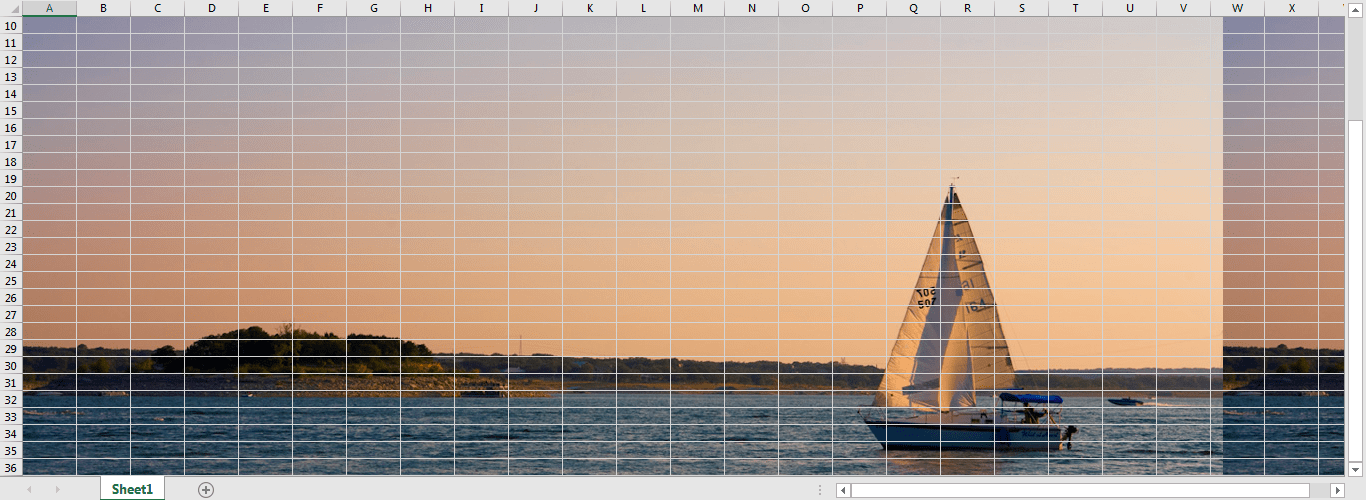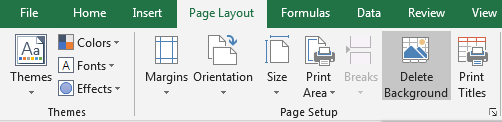# How to Insert Picture as Background in Excel

Sometimes we need to insert a picture for worksheet background in excel per some requests. This article will show you the method to insert picture as background image and we will also show you the method of delete background image.

## Method: Insert Picture as Background

Insert a picture into worksheet is very easy, just click Insert->Illustrations->Picture, then you can select a picture from different paths in your computer.But if we want to set image or picture as worksheet background, we may do more operations.

Step 1: Click Page Layout in ribbon, then click Background in Page Setup group.Step 2: There are three ways to select pictures. Select one path to choose your picture.Step 3: If you pick up a picture from your computer, just click Browse next to ‘From a file’, then your picture stage will be loaded.Step 4: Choose a picture. You will find Open button is changed to Insert button after choosing picture. Click Insert to insert selected picture.Step 5: Verify that this picture is displayed for worksheet background.Comment:

You can also insert picture for background via the other two ways. Make sure that website is connected properly if you choose picture from Bing Image Search or OneDrive.

## Method: Delete Background Picture

Just click on Page Layout->Delete Background, then background picture is deleted.Related Posts

Calculate Years Between Dates In Ms Excel

If you are an avid Ms Excel user, then you might have come across a task in which you needed to calculate the years between the dates; you might take it easy and do this task manually, which is also ...

Calculate Number of Hours between Two Times

Calculating the difference between two times might be a valuable statistic for subsequent computations or averages, whether you're producing a time sheet for staff or recording personal exercises. While Excel has a plethora of complex functions, including date and time ...

Calculate Loan Interest in Given Year

When you borrow money, you are supposed to repay it gradually. Lenders, on the other hand, want to be compensated for their services and the risk they incur by lending you money. That is, you will not just repay the ...

Calculate Interest Rate for Loan

The interest rate is the fee charged by a lender to a borrower and is expressed as a percentage of the principal—the lent amount. The interest rate on a loan is often expressed as an annual percentage rate, abbreviated as ...

Calculate Interest for Given Period

Using the IPMT function in Excel, we can compute the interest payment on any loan. This step-by-step tutorial will guide Excel users of all skill levels through the process to calculate interest for given period. Finally, the formula: =IPMT(B3/12,1,B5,-B2) The ...

How To Use Excel GCD Function

This post will guide you how to use Excel GCD function with syntax and examples in Microsoft excel. Description The Excel GCD function Returns the greatest common divisor of two or more integers. So you can use the GCD function ...

Calculate A Ratio From Two Numbers In Excel

In elementary mathematics, a ratio is a connection or comparison between two or more integers. For example, ratios are often expressed as ":" to demonstrate the relationship between two numbers. You would think that manually calculating a ratio from two ...

How To Use Excel RRI Function

This post will guide you how to use Excel RRI function with syntax and examples in Microsoft excel. Description The Excel RRI function Returns an equivalent interest rate for the growth of an investment. So you can use the RRI ...

CAGR Formula Examples in Excel

CAGR in Excel is a formula that calculates the compound annual growth rate for any invested amount over the specified years or timeframe. Although there is no direct function in Excel that can help us identify the CAGR value, there ...

Build Hyperlink With VLOOKUP in Excel

You might have come across a task in which you were assigned to build hyperlinks, which seems very easy, and if you are new to excel or don't have enough experience with it, then you might wonder about doing this ...

Sidebar